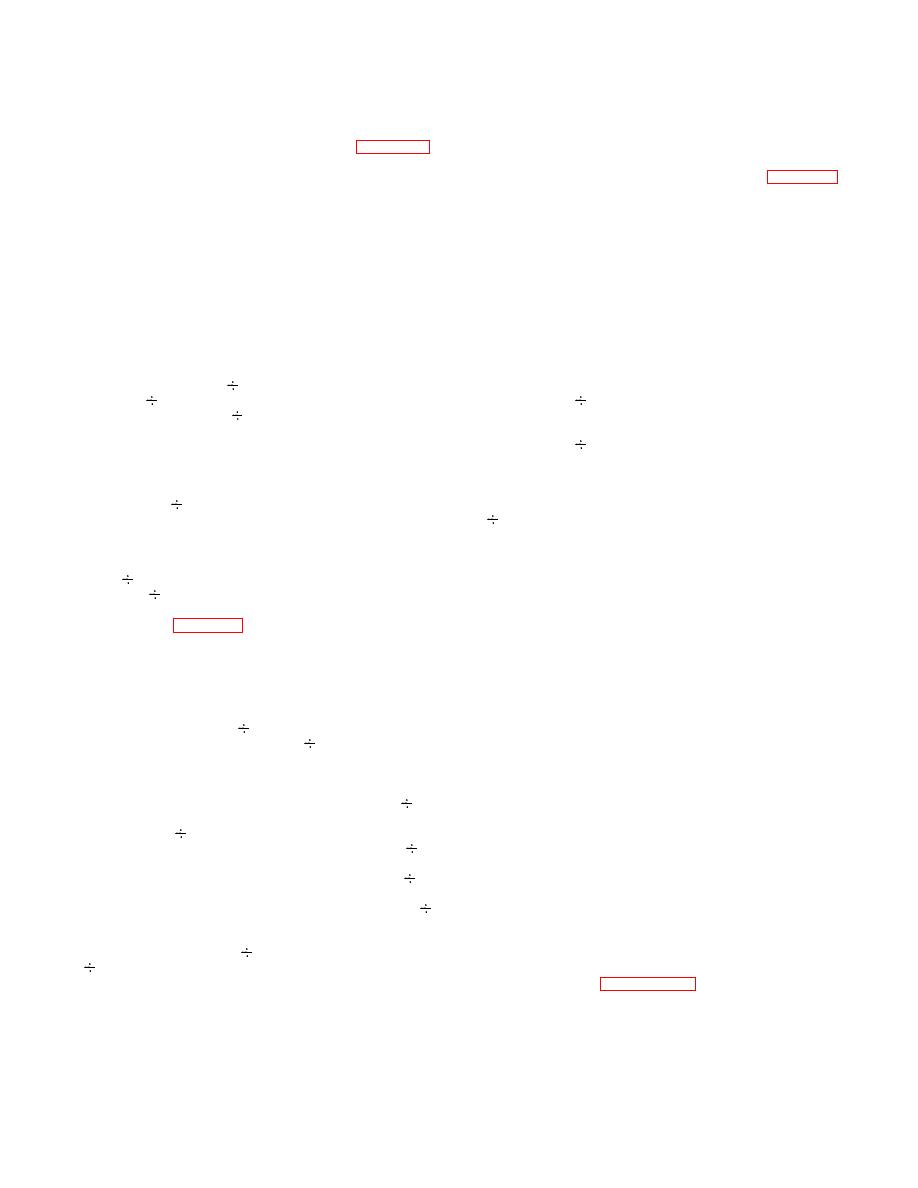Click here to make tpub.com your Home Page Page Title: FREQUENCY SYNTHESIZER MODULE A2. Back | Up | NextWeb www.tpub.comHome

Information Categories
Aerographer
Automotive
Aviation
Construction
Diving
Draftsman
Engineering
Electronics
Food and Cooking
Logistics
Math
Medical
Music
Nuclear Fundamentals
Photography
ReligionTO 31R2-2GRC171-2
TM 11-5820-815-14
NAVELEX 0967-LP-544-5010
power amplifier module A8 and to receive injection
to start a new cycle. For the complete cycle, the number
frequency to receiver rf module A3. Refer to figure 4-1.
of pulses counted by the variable divider is 2 [(41)(A) +
The p11 frequency synthesizer operates as follows: The
variable divider divides the output frequency (FO) by N
40 (Np - A)]. (In the example frequency of figure 4-1, the
and applies the resulting frequency (FV) to the phase
number of pulses counted is 2 [(41)(35)- 40(325-35)] or
detector.  The phase detector compares FV in both
26.070.) The total number of pulses counted is the
frequency and phase to the reference frequency (FR).
division ratio (N) of the variable divider.  The system
The frequency or phase difference between the two
equation for the phase- lock loop is, therefore, given by
signals produces an error signal which is filtered and
the following equation:
applied to the voltage-controlled oscillator (vco). The vco
changes frequency until FV equals FR.  Phase lock
FO = (FR)(2) [41 (A) + 40 (Np - A)]
occurs when FV equals FR and their phase difference is
Where:
constant, The system equation is FO = FR x N.
4-62.  The variable divider functions as a counter
FR = reference frequency
where one output pulse is generated for every N input
pulse. The variable divider consists of a divide-by-2 fixed
prescaler, a divide-by-Np ( Np) programmable counter, a
A = preset count set into
divide-by-A ( A) programmable counter, and a 2-
A programmable counter
modulus prescaler. The  A programmable counter is
programmed (preset to a certain count) by the three least
Np = preset count set into
significant digits of the radio control while the -Np
Np programmable counter
programmable counter is programmed by the three most
significant  digits  of  the  radio  control  and  the
transmit/receive key line.  The 2-modulus prescaler is
4-64.  When operating in the receive mode, the
controlled by the  A programmable counter to divide by
transmit/receive key line causes the division ratio of the
either 40 or 41.
Np programmable counter to decrease by 30.  This
causes  a  30-MHz decrease  in  the  fre-  quency
4-63.  In the 2-modulus prescaling technique, fre-
synthesizer output frequency.  When mixed with the
quency division occurs as follows: At the beginning of a
received signal, the difference results in the 30-MHz if
cycle, the  A programmable counter is preset to a count
frequency.
of A and the  Np programmable counter to a count of
Np as determined by the radio control. (For the example
4-65.  The condition for phase lock occurs when the
frequency given in figure 4-1, A is 35 and Np is 325.) The
phase difference between the variable divider output and
2-modulus prescaler divides the output of the fixed
frequency reference output becomes constant at a value
prescaler by 41 to produce one output pulse for every 41
necessary to produce a vco frequency that when divided
input pulses (82 cycles of FO). The output pulse causes
by N produces 12.5 kHz at the phase detector. The
the preset count of the -A programmable counter and
output frequency of the variable divider locks onto and
+Np program- mable counter to decrement by 1. (These
tracks the phase of the reference frequency. The phase
counters count down from the preset count to zero.) This
difference between the variable divider output and the
process continues until the  A programmable counter is
reference frequency produces a correc- tion voltage at
decremented to zero.  When the
A programmable
the input to the vco. The level of this correction voltage
counter reaches zero count, it feeds back a control signal
is such that it will pull the vco frequency in a direction that
to the 2-modulus prescaler to change its division ratio
will cause the variable divider output to phase-track the
from 41 to 40. To this point, the total number of pulses
reference frequency. Thus, for each setting of the radio
(cycles of FO) counted is 2 x 41 x A. Since the  Np
control, a digital code presets the variable count to an
programmable counter decrements by the same number
integral number (N) representing a desired out put
of counts as the  A programmable counter, the count
frequency. For each desired output frequency there is a
remaining in the -. Np programmable counter is Np  A.
corresponding unique phase difference and tuning
As  the  cycle  continues,  the  output  of  the  2-
voltage to the vco. At each tuned condition the variable
modulusprescaler continues to decrement the
Np
divider output is phase locked to the reference frequency
programmable counter except now it only requires 40
and the two frequencies are equal to 12.5 kHz.  The
input pulses to produce one output pulse. When the
output fre- quency (vco frequency) is therefore equal to
Np programmable counter is decremented to zero, it
the following:
produces one output pulse to the phase detector and at
the same time resets the  Np programmable counter
vco frequency = N x 12.5 kHz
and  A programmable counter to the preset count of Np
and A, respectively.
4-66.  Refer to figure FO-5 for a block diagram of
frequency synthesizer module A2. The low-band,
4-11Integrated Publishing, Inc. - A (SDVOSB) Service Disabled Veteran Owned Small Business